# MathQA

## Dataset, Annotation, Validation and Examples

Our dataset is gathered by using a new representation language to annotate over the AQuA-RAT dataset. AQuA-RAT has provided the questions, options, rationale, and the correct options.

•   Question: A train running at the speed of 48 km / hr crosses a pole in 9 seconds . what is the length of the train ?
•   Rationale: Speed = ( 48 x 5 / 18 ) m / sec = ( 40 / 3 ) m / sec . length of the train = ( speed x time ) . length of the train = ( 40 / 3 x 9 ) m = 120 m . answer is c .
•   Options: a ) 140 , b ) 130 , c ) 120 , d ) 170 , e ) 160
•   Correct Option is: C

The rationales are noisy, incomplete and sometimes incorrect. We correct these rationales and provide stepwise solutions for a portion of AQuA-RAT.

•   Our Annotated Formula: multiply(divide(multiply(48, const_1000), const_3600), 9)

## Annotations

### Annotation Scheme Explanation

We have designed an annotation platform through the Figure Eight platform. We have defined a representation language that can capture the steps that are necessary for finding the solution to the problem. Each step in our final program is the predefined operation and its arguments, which can be chosen from the problems, a list of constants or previous calculations. Here is the overview of our job:

• Problem text is shown to the contributors.
• Based on the category of the problem, related operations are shown to the contributor.
• Contributors can see a hint containing the formula, argument and explanation by hovering over operations.
• After selecting an operation, a list of valid arguments are shown to the contributor to choose from. When the arguments list is complete, the output value of the operation is calculated and added to the valid arguments list.
• This process will continue until the operation values are close to the final answer with a error margin.
• We record the contributor’s actions in the form of a line of math expressions.

Figure eight provides unique features that help us achieve data that is clean, reliable and accurate. The task of annotating math word problem is especially complicated and time consuming task. The points that should considered are :

• Accuracy of the solution : To ensure accuracy we have defined several test questions which will be shown to contributors randomly and they should maintain an accuracy above the threshold. We constantly monitored contributors’ answers to a quality question to allow different variations for a solution.
• Submission validation : Having a final correct answer lets us check the calculation result of a contributor-provided program. The solution can only be submitted if the final answer matches the correct answer and the contributor used at least one value from problem.

#### Validation Scheme Explanation

The results of the annotation task are not all correct. There are cases that a logically wrong program would get us to the final answer. Therefore we designed a validation task. Here are the steps for that task:

• The contributor will be presented with the following components: The problem, the rationale, and the math expression that has been annotated as the solution.
• By clicking over every part of the expression they can see the calculation result if they want to compare the number with the numbers that are mentioned in the rationale.
• If the annotation is aligned and correct with rationale they should choose “yes” ; Otherwise they should choose “no”.

The quality of the validation task is also set by designing the quality questions.

##### • amini91@cs.washington.edu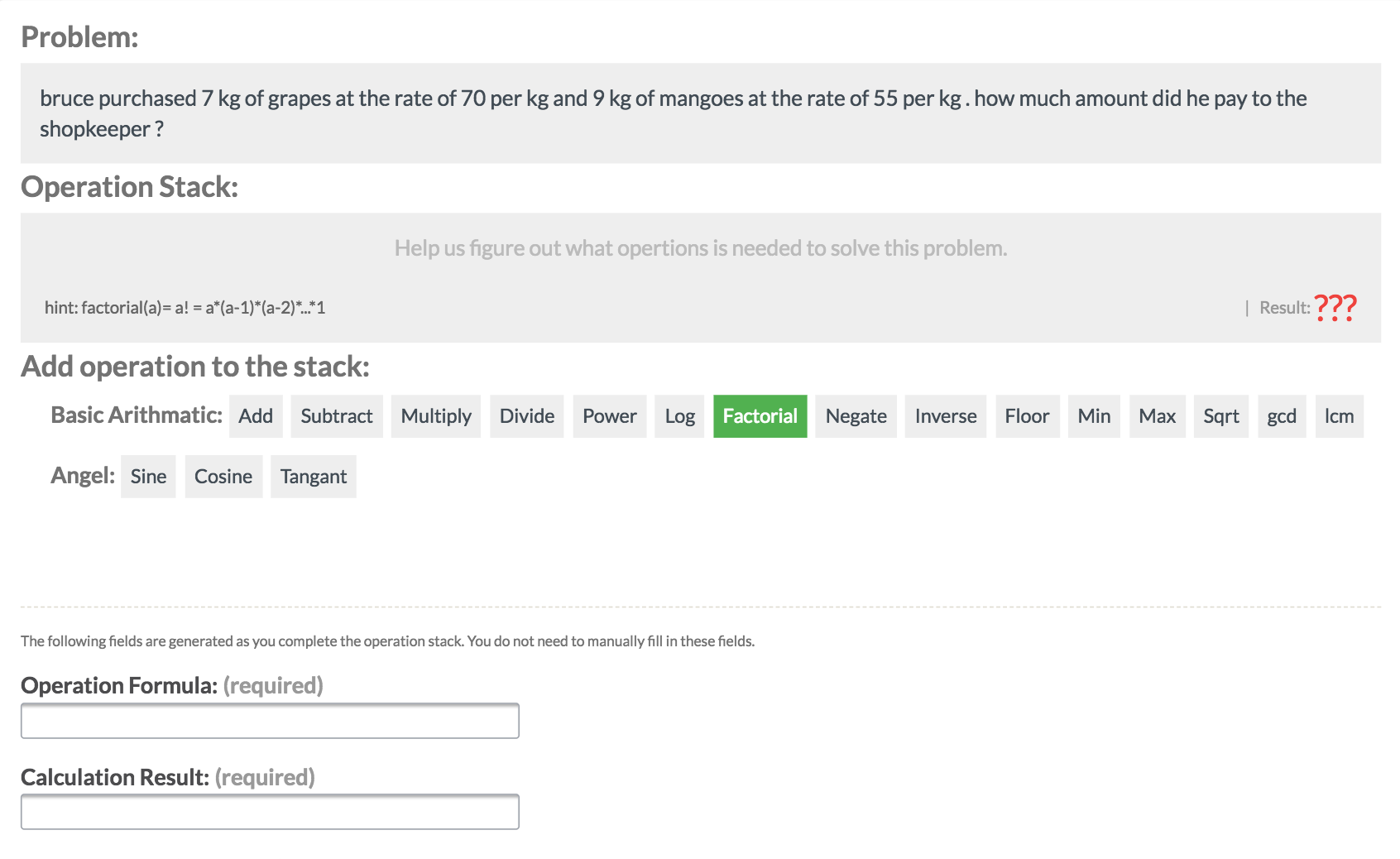By hovering over the opration the hind will be shown in the operation stack section.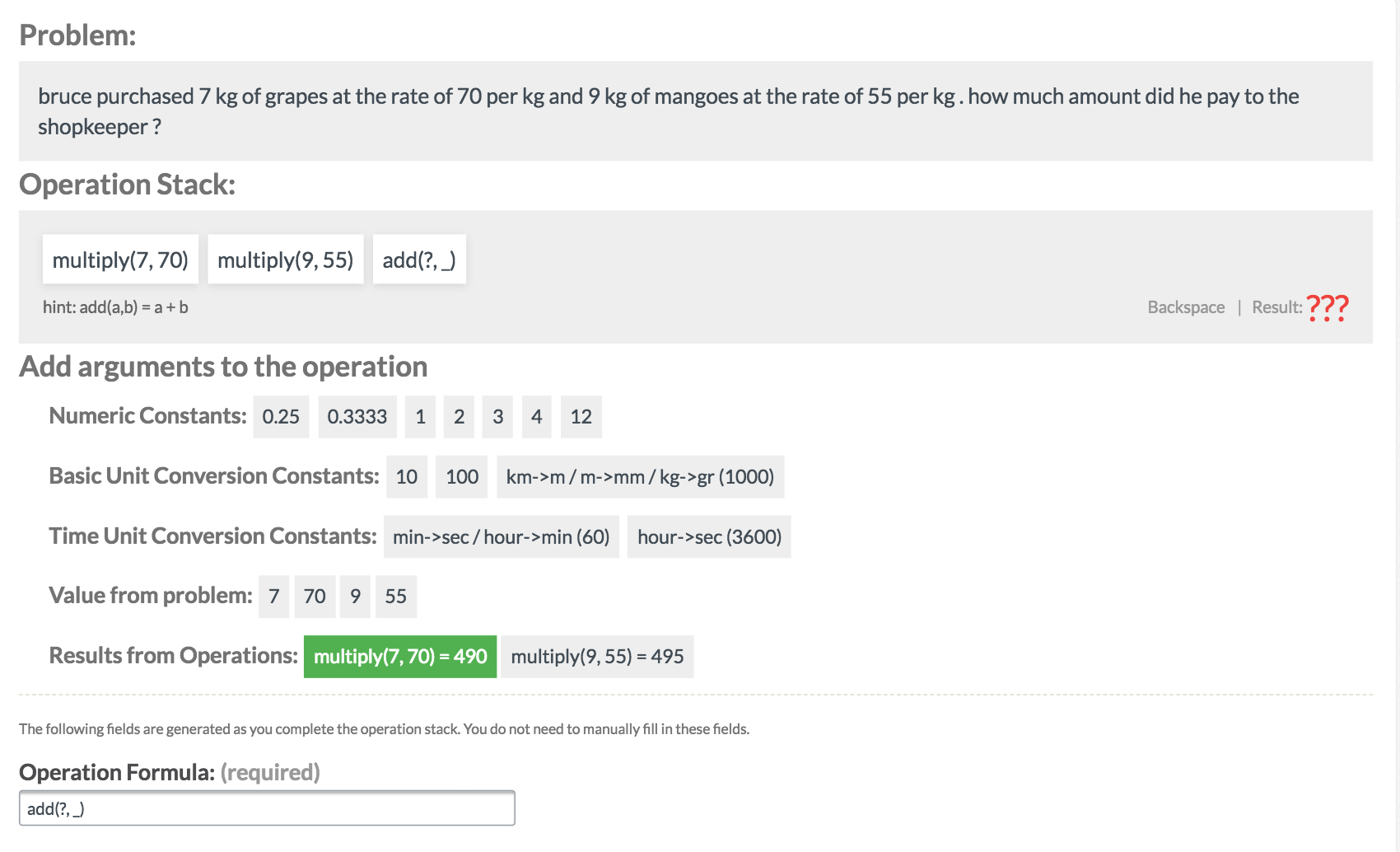The previous calculations are added to the list of valid arguments.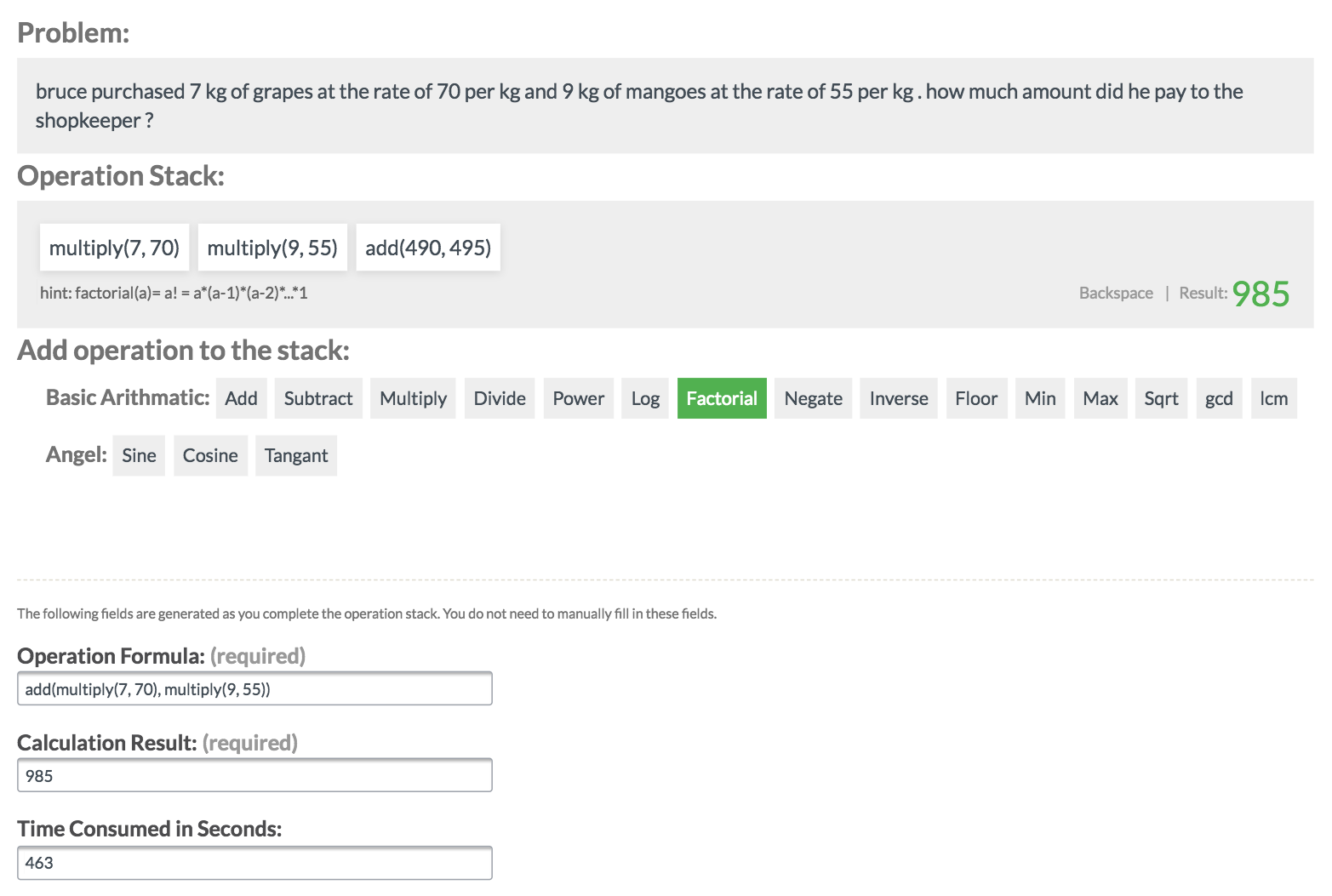The final answer is acceptable when the currect calculation result is the same as the correct option and at least one number from problem is used. The Result would automatically copied to the requiredd result field which let the contributors to submit their results.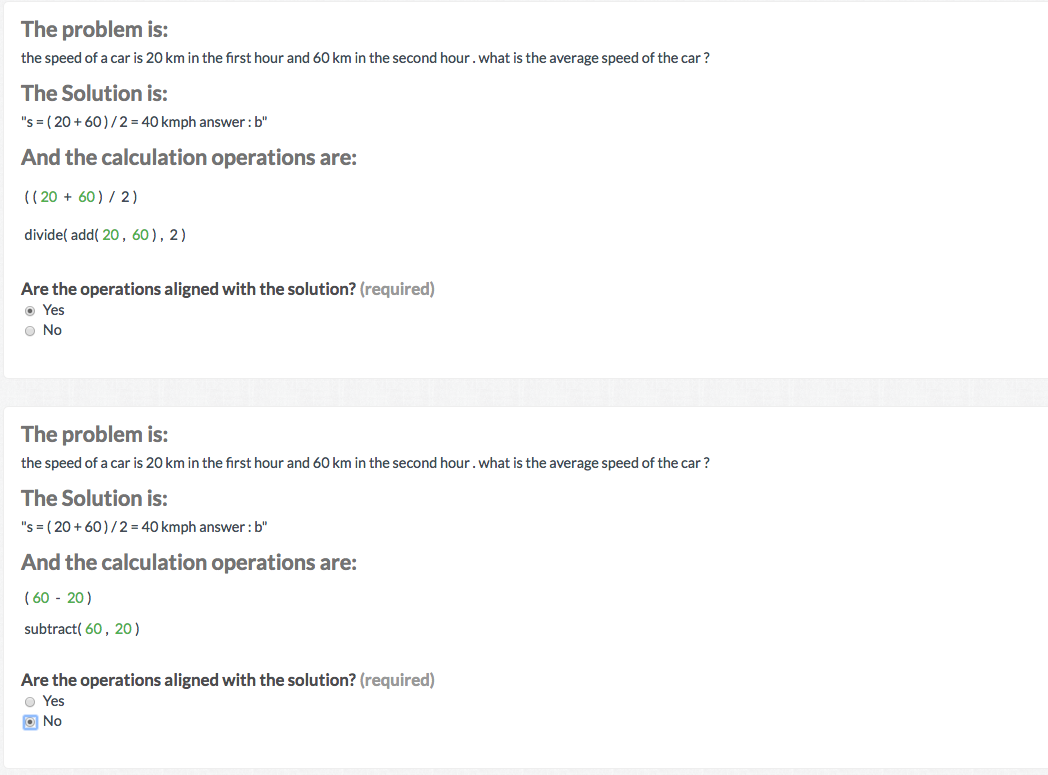Two paths for a problems, both resulting in a same number, but the one on the buttom is logically wrong.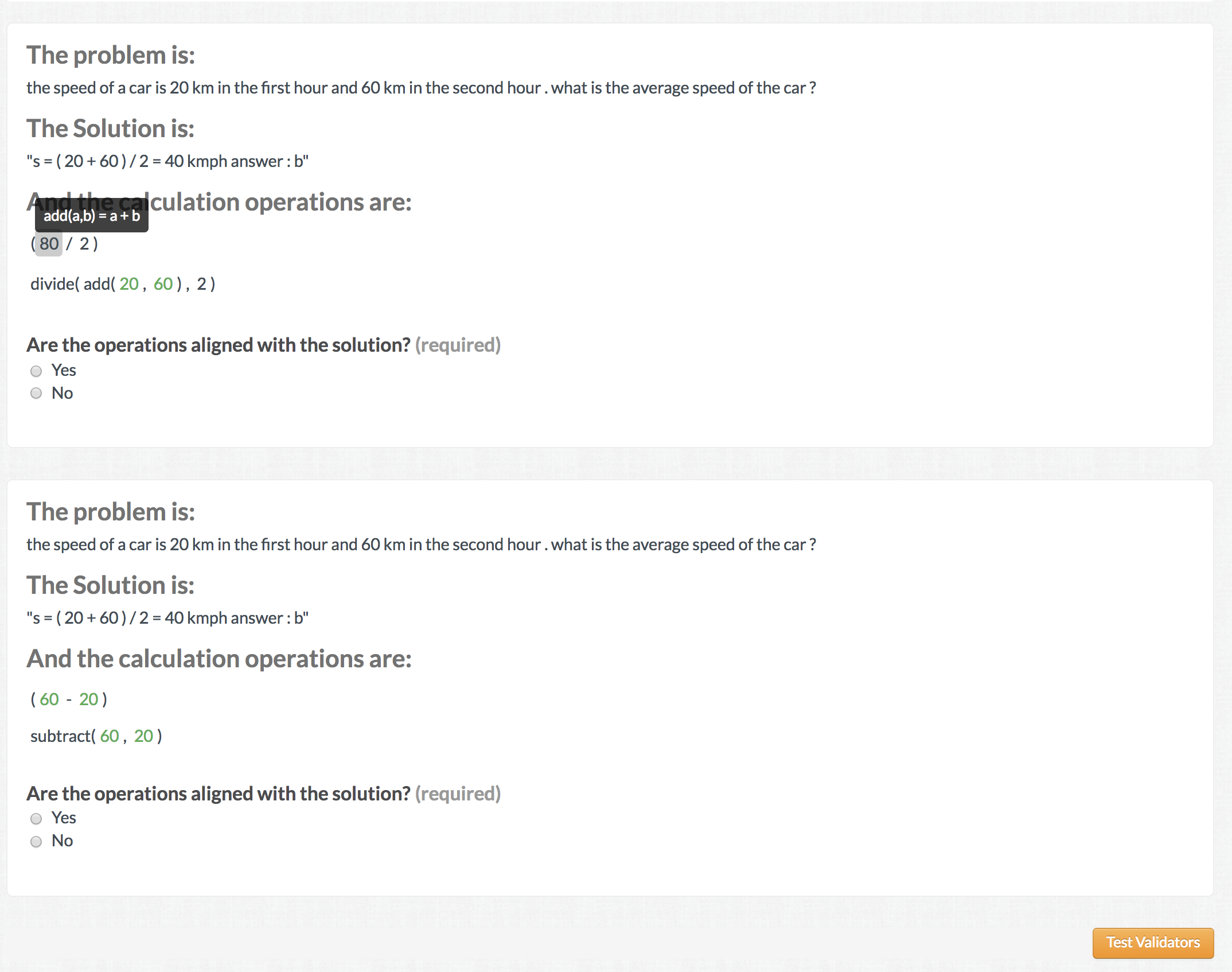By clicking on portion of experssion contributors can see the result for that part of expression.